Electron. J. Diff. Eqns., Vol. 2003(2003), No. 104, pp. 1-23.

### A linear functional differential equation with distributions in the input Vadim Z. Tsalyuk

Abstract:
This paper studies the functional differential equation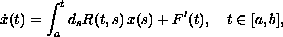where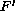is a generalized derivative, and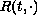and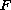are functions of bounded variation. A solution is defined by the difference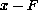being absolutely continuous and satisfying the inclusion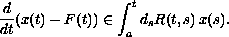Here, the integral in the right is the multivalued Stieltjes integral presented in  (in this article we review and extend the results in ). We show that the solution set for the initial-value problem is nonempty, compact, and convex. A solutionis said to have memory if there exists the function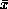such that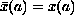,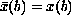,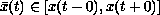for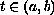, and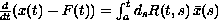, where Lebesgue-Stieltjes integral is used. We show that such solutions form a nonempty, compact, and convex set. It is shown that solutions with memory obey the Cauchy-type formula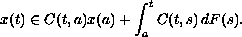Submitted June 14, 2003. Published October 13, 2003.
Math Subject Classifications: 26A42, 28B20, 34A60.
Key Words: Stieltjes integral, function of bounded variation, multivalued integral, linear functional differential equation.

Show me the PDF file (285K), TEX file, and other files for this article.Vadim Z. Tsalyuk Mathematics Department Kuban State University Stavropol'skaya 149, Krasnodar 350040, Russia email: vts@math.kubsu.ru http://public.kubsu.ru/vts (in Russian)